CipherJan 15, 2017

Saturn V rocket used for all Apollo missionsSaturn V is still the tallest, heaviest and most powerful rocket ever used by NASA,  it was used for all Apollo Missions and launch of Skylab

All of the launches were from "LC-39" - L = 12th letter, 1+2= 3,  C = 3rd letter,  which makes LC = 33
Total of 13 launches, payload capacity of 310,000 lb

Launched on 11/9/1967 - 11+9+1+9+6+7 - 43 (13 x 31 = 403)Speed of Saturn is 9.69 km/s

"Star of David" = 119 (Ordinal) ,"God Saturn" = 119 (O) , "Saturn" = 45+1+20+21+18+14 = 119 (Francis Bacon), Milky Way = 119, All Seeing Eye = 119"Saturn V" = 93 + 22 = 115 (Ordinal)
"Saturn" = 90+1+100+200+80+40 = 511 (Jewish)77th triangular number is 3003 (Christ - 77, dead at 33)

"Saturn Five" = 21 + 24 = 45 (Reduced) - Magic square of Saturn sums to 45, also Saturn is supposedly 4.5 billion years old

"Saturn Five" = 93 + 42 = 135 (Ordinal), it is comparable to 'N-1' rocket, "N-One" = 21, "N-one" = 40 + 50+40+5 = 135 (Jewish)1/10 -  rocket is 110 meters tall (1/10/62 = 1+10+62 = 73, the 21st prime number, Saturn = 21)
"C-Five" = 3 + 6+9+22+5 = 45 (Ordinal)
"Saturn Five" = 21 + 24 = 45 (Reduced)

Designed for 90,000 pound (41,000 kg) payload capacity... 13th prime is 41
"Ninety" = 5+9+5+5+2+7 = 33 (Reduced)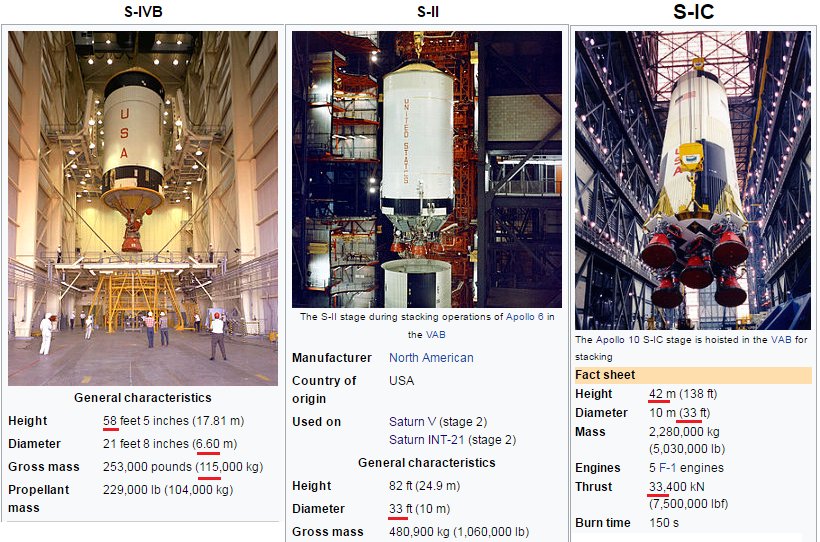They always build all their stuff by their favorite numbers, I won't get into dimensions too much
"S-IVB" = 100 + 9+400+2 = 511 (English), Saturn = 511 (Jewish)
"S-IVB" = 1 + 9+4+2 = 16  - "Sixteen" = 1+9+6+2+5+5+5 = 33  - English gematria of Sixteen is also interesting - "Sixteen" = 100+9+600+200+5+5+50 = 969 (English)

S-IVB is 58 feet high and has mass of 115,000 kg
"Fifty-eight" = 6+9+6+20+25 + 5+9+7+8+20 = 115 (Ordinal)

"S-II" = 45 + 35+35 = 115 (Francis Bacon), was also used on 'Saturn INT-21' rocket... Very obvious, "Saturn" = 1+1+2+3+9+5 = 21

Interestingly there was an episode of Star Trek where they featured Saturn V rocket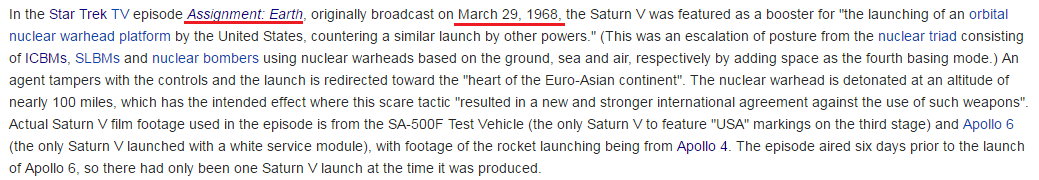First flight of Saturn V was on 11/9The episode of Star Trek was aired on 3/29/1968 - 3+29+19+68 = 119
It's cute how it is - Season 2, Episode 26
"Two hundred twenty six" = 13 + 38 + 26 + 16 = 93 (Reduced) like Saturn
- Story by - "Art Wallace" = 12 + 21 = 33 (Reduced)
"Gene Roddenberry" = 22 + 65 = 87 (Reduced) - first flight of Saturn V was on a date numerology of 87 (11+9+67 = 87)
- Directed by - "Marc Daniels" = 134 + 199 = 333 (English)
"Marc Daniels" = 45 / 99 (Thirteen = 45/99)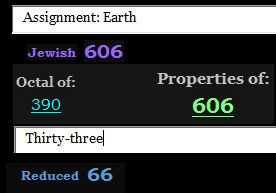On last flight Saturn V launched Skylab 1 into orbit, in the year 1973 , 21st prime is 73 (Saturn = 21)

5/14/1973 - 5+14+1+9+7+3 = 39 (May = 13+1+25 = 39) - 39 books in the Old Testament, Saturn = 93 (God of the Old Testament), first Manned Moon (Apollo 11) mission was launched on 7/16/1969 (39 date numerology)
5/14/1973 - 5+1+4+1+9+7+3 = 30 (prime 113, Universe = 113, Mainstream = 113, Space Suit = 113, Explorer = 113, Green Screen = 113)
5/14/1973 - 5+14+19+73 = 111

Skylab informationLength - 82.4 feet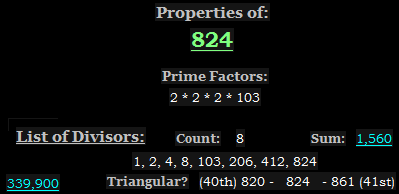Thirty-three = 156/66 (Diameter of Skylab - 6.6 meters)

Width - 55.8 feet
"Saturn" = 114+6+120+126+108+84 = 558 (Sumerian)

Height - 11.1 m (36.3 feet, Saturn V is 363 feet tall)
Skylab was launched on a date with 111 numerology, Apollo 11 was also launched on a date with 111 numerology (7+16+19+69 = 111)

Orbital period of Skylab couldn't be better either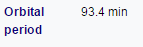Saturn = 93 (Ordinal)